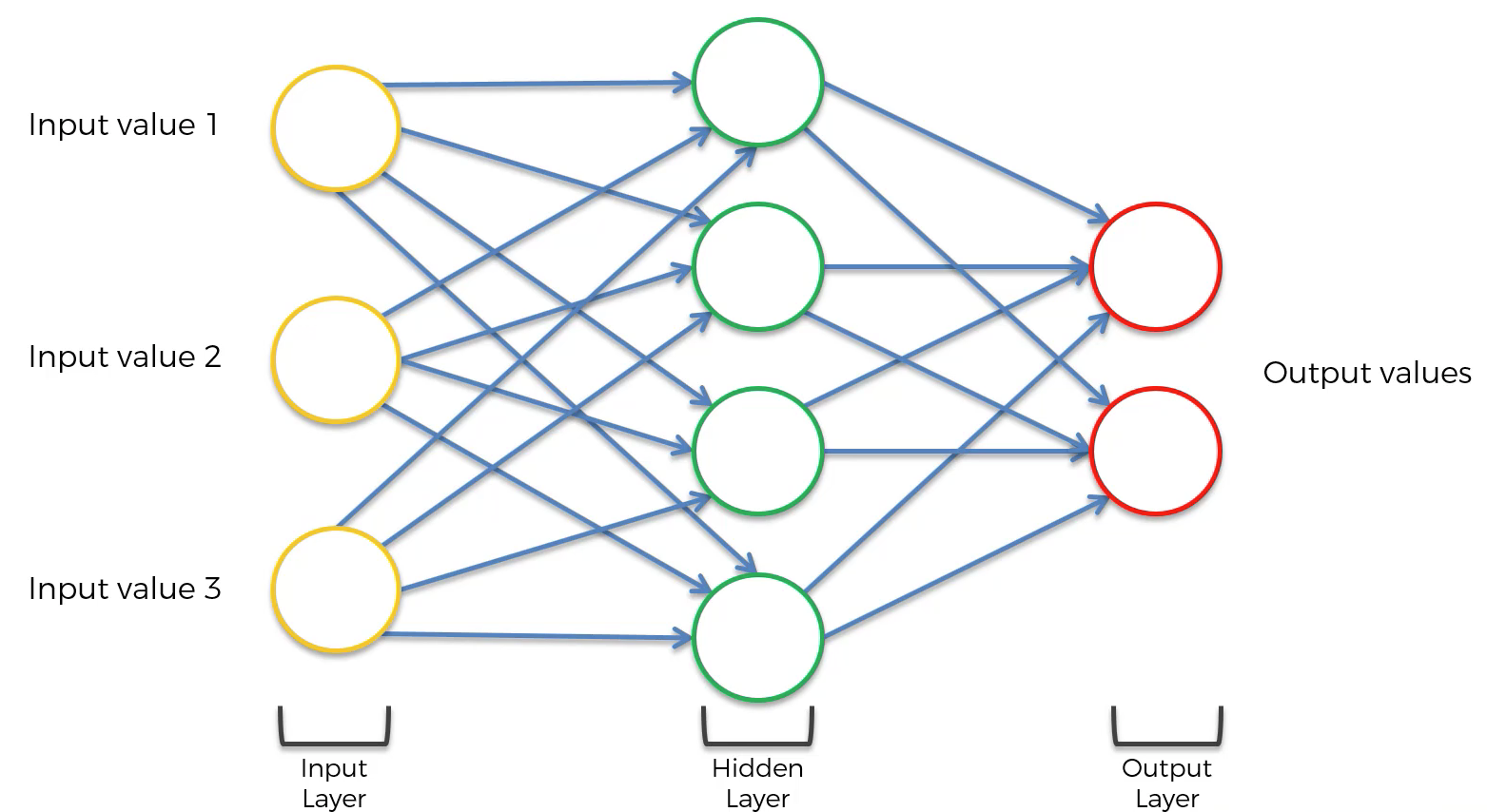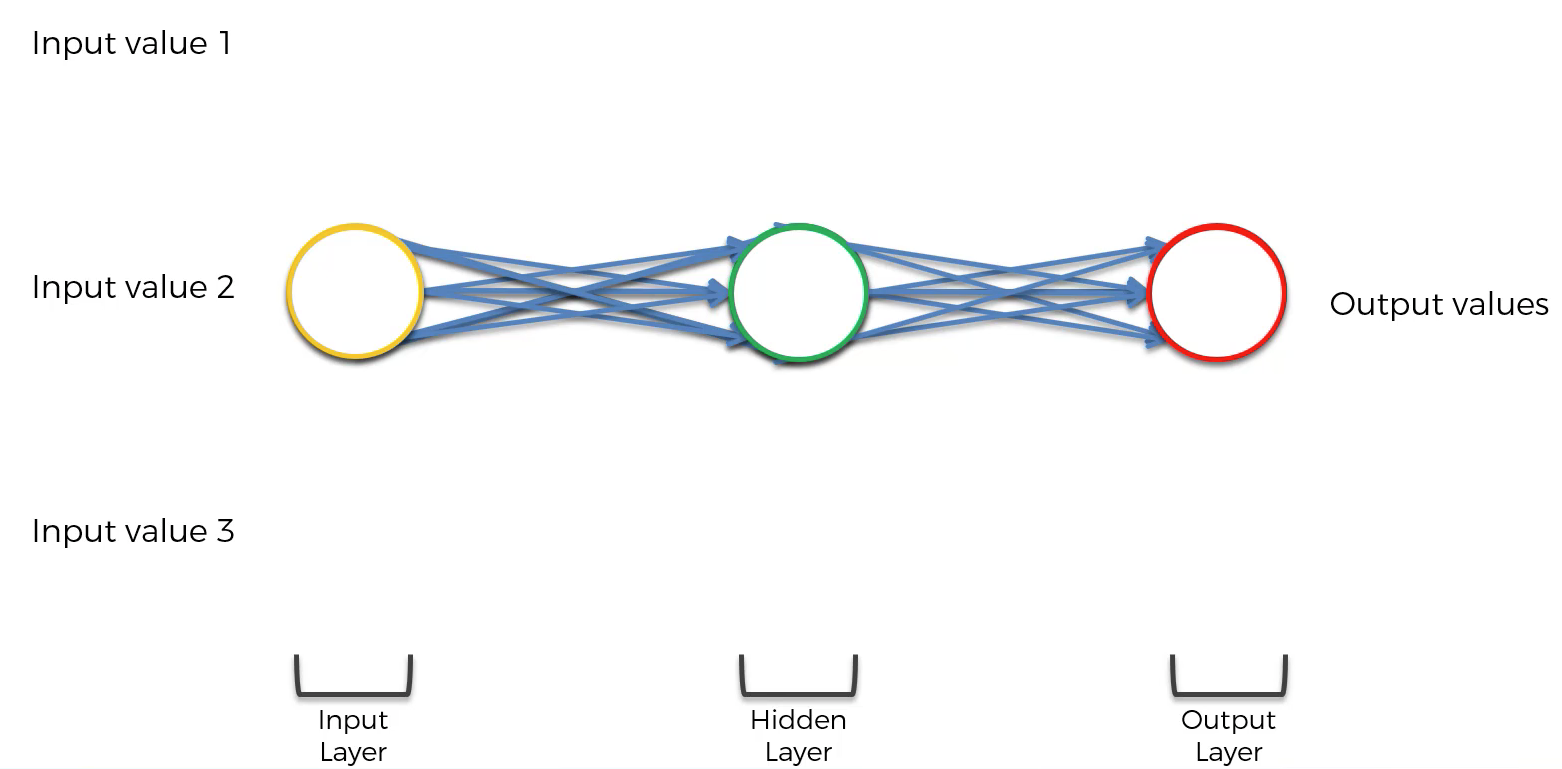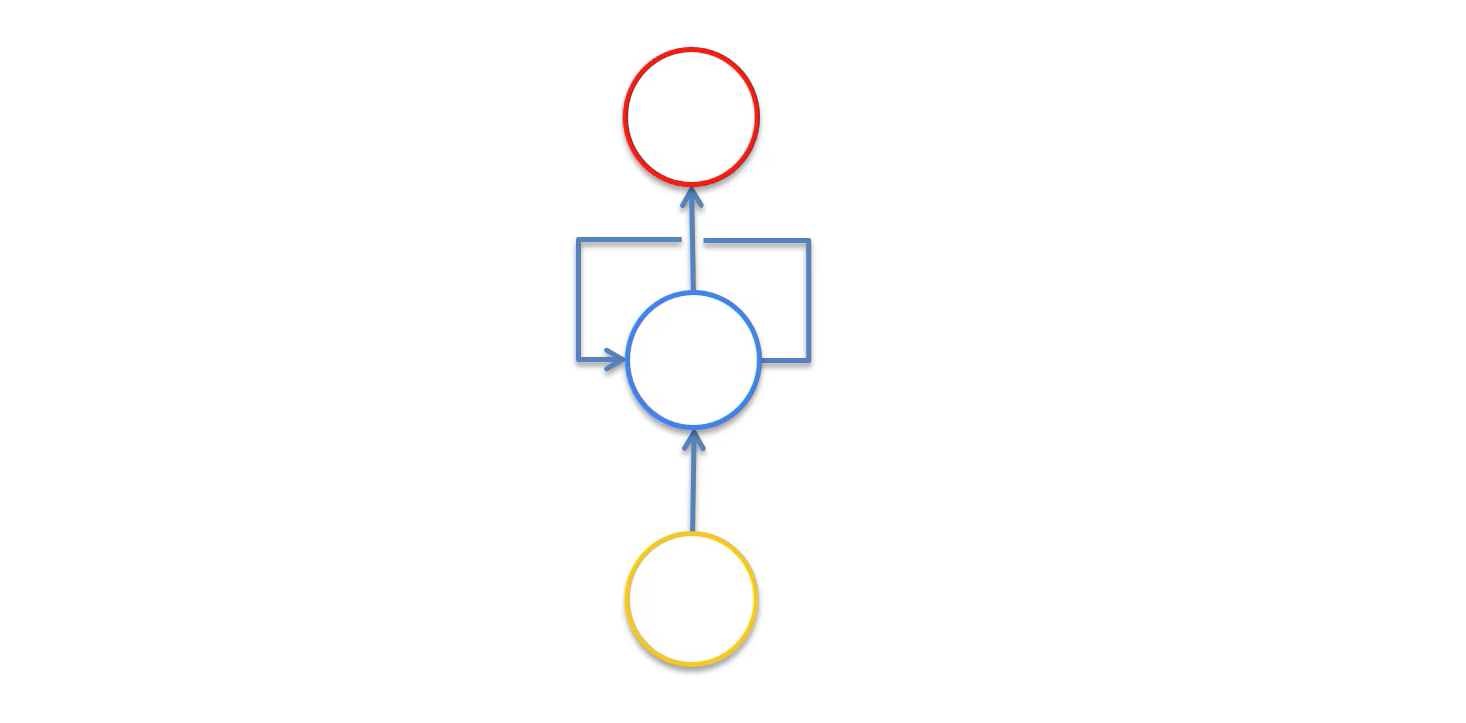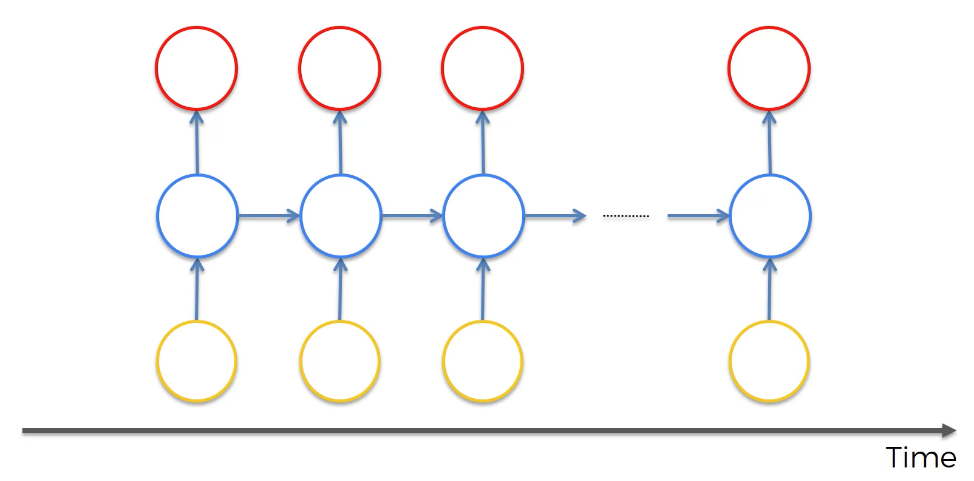# The Intuition of Recurrent Neural Networks

Hey - Nick here! This page is a free excerpt from my \$199 course Python for Finance, which is 50% off for the next 50 students.

So far in this course, you have learned about the following types of neural networks:

The next type of neural network that we'll discuss is a recurrent neural network.

This tutorial will begin our discussion of recurrent neural networks by discussing the intuition behind recurrent neural networks.

## The Types of Problems Solved By Recurrent Neural Networks

Although we have not explicitly discussed it yet, there are generally broad swathes of problems that each type of neural network is designed to solve:

In the case of recurrent neural networks, they are typically used to solve time series analysis problems.

Each of these three types of neural networks (artificial, convolutional, and recurrent) are used to solve supervised machine learning problems.

## Mapping Neural Networks to Parts of the Human Brain

As you'll recall, neural networks were designed to mimic the human brain. This is true for both their construction (both the brain and neural networks are composed of neurons) and their function (they are both used to make decisions and predictions).

The three main parts of the brain are:

• The cerebrum
• The brainstem
• The cerebellum

Arguably the most important part of the brain is the cerebrum. It contains four lobes:

• The frontal lobe
• The parietal lobe
• The temporal lobe
• The occipital lobe

The main innovation that neural networks contain is the idea of weights.

Said differently, the most important characteristic of the brain that neural networks have mimicked is the ability to learn from other neurons.

The ability of a neural network to change its weights through each epoch of its training stage is similar to the long-term memory that is seen in humans (and other animals).

The temporal lobe is the part of the brain that is associated with long-term memory. Separately, the artificial neural network was the first type of neural network that had this long-term memory property. In this sense, many researchers have compared artificial neural networks with the temporal lobe of the human brain.

Similarly, the occipital lobe is the component of the brain that powers our vision. Since convolutional neural networks are typically used to solve computer vision problems, you could say that they are equivalent to the occipital lobe in the brain.

As mentioned, recurrent neural networks are used to solve time series problems. They can learn from events that have happened in recent previous iterations of their training stage. In this way, they are often compared to the frontal lobe of the brain - which powers our short-term memory.

To summarize, researchers often pair each of the three neural nets with the following parts of the brain:

• Artificial neural networks: the temporal lobe
• Convolutional neural networks: the occipital lobe
• Recurrent neural networks: the frontal lobe

## The Composition of a Recurrent Neural Network

Let's now discuss the composition of a recurrent neural network. First, recall that the composition of a basic neural network has the following appearance:The first modification that needs to be made to this neural network is that each layer of the network should be squashed together, like this:Then, three more modifications need to be made:

• The neural network's neuron synapses need to be simplified to a single line
• The entire neural network needs to be rotated 90 degrees
• A loop needs to be generated around the hidden layer of the neural net

The neural network will now have the following appearance:That line that circles the hidden layer of the recurrent neural network is called the temporal loop. It is used to indicate that the hidden layer not only generates an output, but that output is fed back as the input into the same layer.

A visualization is helpful in understanding this. As you can see in the following image, the hidden layer used on a specific observation of a data set is not only used to generate an output for that observation, but it is also used to train the hidden layer of the next observation.This property of one observation helping to train the next observation is why recurrent neural networks are so useful in solving time series analysis problems.

## Final Thoughts

In this tutorial, you had your first introduction to recurrent neural networks. More specifically, we discussed the intuition behind recurrent neural networks.

Here is a brief summary of what we discussed in this tutorial:

• The types of problems solved by recurrent neural networks
• The relationships between the different parts of the brain and the different neural networks we've studied in this course
• The composition of a recurrent neural network and how each hidden layer can be used to help train the hidden layer from the next observation in the data set